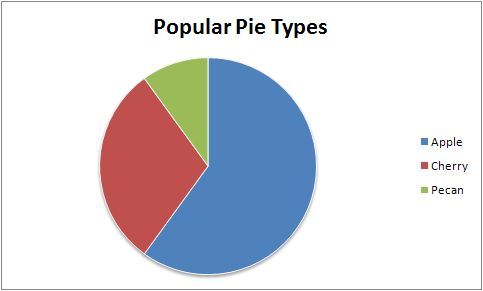and 1 contributors

# NAME

Pie - A class for writing Excel Pie charts.

# SYNOPSIS

To create a simple Excel file with a Pie chart using Excel::Writer::XLSX:

``````    #!/usr/bin/perl

use strict;
use warnings;
use Excel::Writer::XLSX;

my \$workbook  = Excel::Writer::XLSX->new( 'chart.xlsx' );

my \$chart     = \$workbook->add_chart( type => 'pie' );

# Configure the chart.
categories => '=Sheet1!\$A\$2:\$A\$7',
values     => '=Sheet1!\$B\$2:\$B\$7',
);

# Add the worksheet data the chart refers to.
my \$data = [
[ 'Category', 2, 3, 4, 5, 6, 7 ],
[ 'Value',    1, 4, 5, 2, 1, 5 ],
];

\$worksheet->write( 'A1', \$data );

__END__``````

# DESCRIPTION

This module implements Pie charts for Excel::Writer::XLSX. The chart object is created via the Workbook `add_chart()` method:

``    my \$chart = \$workbook->add_chart( type => 'pie' );``

Once the object is created it can be configured via the following methods that are common to all chart classes:

``````    \$chart->add_series();
\$chart->set_title();``````

These methods are explained in detail in Excel::Writer::XLSX::Chart. Class specific methods or settings, if any, are explained below.

# Pie Chart Methods

## set_rotation()

The `set_rotation()` method is used to set the rotation of the first segment of a Pie/Doughnut chart. This has the effect of rotating the entire chart:

``    \$chart->set_rotation( 90 );``

The angle of rotation must be `0 <= rotation <= 360`.

## User defined colors

It is possible to define chart colors for most types of Excel::Writer::XLSX charts via the add_series() method. However, Pie/Doughnut charts are a special case since each segment is represented as a point so it is necessary to assign formatting to each point in the series:

``````    \$chart->add_series(
values => '=Sheet1!\$A\$1:\$A\$3',
points => [
{ fill => { color => '#FF0000' } },
{ fill => { color => '#CC0000' } },
{ fill => { color => '#990000' } },
],
);``````

See the main Excel::Writer::XLSX::Chart documentation for more details.

``````    \$chart->add_series(
name        => 'Pie sales data',
categories  => [ 'Sheet1', 1, 3, 0, 0 ],
values      => [ 'Sheet1', 1, 3, 1, 1 ],
data_labels => {
series_name  => 1,
percentage   => 1,
position     => 'outside_end'
},
);``````

Note: Even when leader lines are turned on they aren't automatically visible in Excel or Excel::Writer::XLSX. Due to an Excel limitation (or design) leader lines only appear if the data label is moved manually or if the data labels are very close and need to be adjusted automatically.

## Unsupported Methods

A Pie chart doesn't have an X or Y axis so the following common chart methods are ignored.

``````    \$chart->set_x_axis();
\$chart->set_y_axis();``````

# EXAMPLE

Here is a complete example that demonstrates most of the available features when creating a chart.

``````    #!/usr/bin/perl

use strict;
use warnings;
use Excel::Writer::XLSX;

my \$workbook  = Excel::Writer::XLSX->new( 'chart_pie.xlsx' );
my \$bold      = \$workbook->add_format( bold => 1 );

# Add the worksheet data that the charts will refer to.
my \$headings = [ 'Category', 'Values' ];
my \$data = [
[ 'Apple', 'Cherry', 'Pecan' ],
[ 60,       30,       10     ],
];

\$worksheet->write( 'A2', \$data );

# Create a new chart object. In this case an embedded chart.
my \$chart = \$workbook->add_chart( type => 'pie', embedded => 1 );

# Configure the series. Note the use of the array ref to define ranges:
# [ \$sheetname, \$row_start, \$row_end, \$col_start, \$col_end ].
name       => 'Pie sales data',
categories => [ 'Sheet1', 1, 3, 0, 0 ],
values     => [ 'Sheet1', 1, 3, 1, 1 ],
);

\$chart->set_title( name => 'Popular Pie Types' );

# Set an Excel chart style. Colors with white outline and shadow.
\$chart->set_style( 10 );

# Insert the chart into the worksheet (with an offset).
\$worksheet->insert_chart( 'C2', \$chart, 25, 10 );

__END__``````

This will produce a chart that looks like this:# AUTHOR

John McNamara jmcnamara@cpan.org